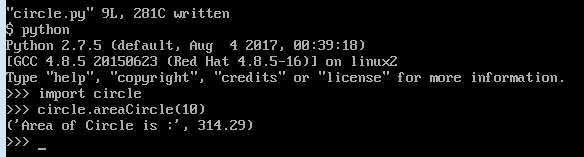### Best Practices for Handling Duplicate Elements in Python ListsHere are three awesome ways that you can use to remove duplicates in a list. These are helpful in resolving your data analytics solutions.  01. Using a Set Convert the list into a set , which automatically removes duplicates due to its unique element nature, and then convert the set back to a list. Solution: original_list = [2, 4, 6, 2, 8, 6, 10] unique_list = list(set(original_list)) 02. Using a Loop Iterate through the original list and append elements to a new list only if they haven't been added before. Solution: original_list = [2, 4, 6, 2, 8, 6, 10] unique_list = [] for item in original_list:     if item not in unique_list:         unique_list.append(item) 03. Using List Comprehension Create a new list using a list comprehension that includes only the elements not already present in the new list. Solution: original_list = [2, 4, 6, 2, 8, 6, 10] unique_list = [] [unique_list.append(item) for item in original_list if item not in unique_list] All three methods will result in uni

# Calculate Circle Area the Logic You Need to write in Python

In Python, you can calculate the circle area easily by using the function. The purpose of Python is to use in data analysis.

You need this logic in many areas. You can use in your present finance projects or new ones. Benefits of function you can re-use the same code number of times.

```       ```
Area of Circle=pi*r*r       ```
```

## Area of Circle Steps Given - You Can do Using Two Methods, Explained Both Methods

I have given steps to calculate the area of a circle using two different methods. First I followed by creating a user-defined function. Next, directly I ran the formula in the interpreter.

### Method -1

#### Steps I have followed to Calculate Area Using Function

1. Logged into Cent Os (Linux)
2. Create .py module
3. Import .py module into Python
4. Execute .py module

I have first logged into CentOS. You can see there '\$'.

#### 2. Creating .py module

• To create ".py" module. You can use the vi editor command.
• You need 'import decimal' to get Decimal values. Else you will get the only integer.

I have given the PWD command. Which tells the present working directory. There you can give ls command. You can see the list of .py modules. Here, you can see two kinds of modules. Those are .py and .pyc.

The .py is our module, which contains a function to calculate the Circle area. The .pyc module is a Python compiled module that will store in the same directory. When you first import the module in Python, the " .Pyc" module will create by Python.

#### 3. Importing the Module from Operating system

• You need to first go to Python using Python command. There, you can give an import module-name.
• During import .py for the module extension not required.

#### 4. Execute Function with Circle area

You can pass a value to function to get the area of a circle.

####The value is rounded to 2 digits. I used round() function.

#### Bottom Line

Steps I used explained to calculate the area using the function. In a similar way you can calculate areas for:

1. Rectangle area
2. Circle perimeter

## Method -2

### How to calculate Circle area using the command line

You can give values directly in the Circle area formula to get area.